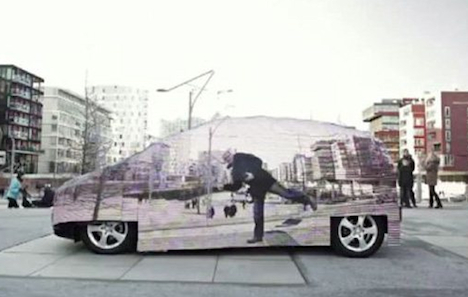## Welcome to H3 Maths

Blog Support for Growing Mathematicians

### Illusions and Mathematics

March5

Mercedes have just created an almost invisible car. A camera relays pictures from the opposite side of the car and this gives you the illusion that you are looking right through the vehicle, which may prove unsafe in some situations?The famous graphic artist, M.C. Escher used the power of illusion in his wonderful drawings. He was a master of the use of symmetry for producing beautiful designs:by posted under Uncategorized | Comments Off on Illusions and Mathematics

#### Post Support

10 x 9 x 8 + (7 + 6) x 5 x 4 x (3 + 2) x 1 = 2020

NCEA Level 2 Algebra Problem. Using the information given, the shaded area = 9, that is:
y(y-8) = 9 –> y.y – 8y – 9 =0
–> (y-9)(y+1) = 0, therefore y = 9 (can’t have a distance of – 1 for the other solution for y)
Using the top and bottom of the rectangle,
x = (y-8)(y+2) = (9-8)(9+2) = 11
but, the left side = (x-4) = 11-4 = 7, but rhs = y+? = 9+?, which is greater than the value of the opp. side??
[I think that the left had side was a mistake and should have read (x+4)?]